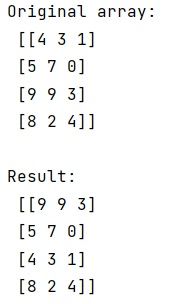# How to swap two rows of a NumPy Array?

Learn, how can we swap two rows of an array using NumPy?
Submitted by Pranit Sharma, on March 27, 2023

Suppose that we are given a 2D numpy array that contains multiple rows (say x, y, p, and q). We need to swap the x and y rows with each other.

## Swapping two rows of an array using NumPy

To swap two rows of a NumPy array, we need to put the index of the rows arr[[x,y]] and then we will assign it with a new order as arr[[y,x]]. This is just a shorthand for arr[[0,2],:]. So this selects the submatrix consisting of all of rows 0 and 2. To interchange columns, you would use a[:, [0, 2]] = a[:, [2, 0]].

Let us understand with the help of an example,

## Python code to swap two rows of a NumPy Array

```# Import numpy
import numpy as np

# Creating a numpy array
arr = np.array([[4,3,1], [5,7,0], [9,9,3], [8,2,4]])

# Display original array
print("Original aarray:\n",arr,"\n")

# Swapping rows 0th and 2nd
arr[[0,2]] = arr[[2,0]]

# Display result
print("Result:\n",arr)
```

### Output